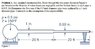# Fluids: Energy equation involving head loss

• reddawg
If P is absolute pressure, then the tank cannot exhaust to atmosphere, since the pressure there > the supposed pressure inside the tank.f

## Homework Statement

See attached image:

## Homework Equations

p/ρg + V^2/2g + z = constant

head loss (major) = f * l/D * V^2/2g

## The Attempt at a Solution

To use the energy equation while incorporating head loss, I need to determine the velocity in each section of pipe. The problem is I don't know how! I do know that the pressure drop from the tank to the atmosphere is equal to the pressure in the tank.

#### Attachments

•problem1.JPG
38 KB · Views: 294
Last edited:
The frictional term has to be properly combined with the bernoulli equation. To get you started, let Q represent volumetric throughput rate. This is what you will be solving for. In terms of Q, what is the velocity of the air in each of the sections. From the ideal gas law, what is the density of the air?

The frictional term has to be properly combined with the bernoulli equation. To get you started, let Q represent volumetric throughput rate. This is what you will be solving for. In terms of Q, what is the velocity of the air in each of the sections. From the ideal gas law, what is the density of the air?
V is equal to the flowrate Q divided by the cross-sectional area of each pipe.

From the ideal gas law, density ρ = p/RT = 6.88*10^-5 slugs/ft^3

V is equal to the flowrate Q divided by the cross-sectional area of each pipe.

From the ideal gas law, density ρ = p/RT = 6.88*10^-5 slugs/ft^3

You have to be careful with units here, especially R for air.

Can you show your calculation of ρ in detail?

You have to be careful with units here, especially R for air.

Can you show your calculation of ρ in detail?
ρ = (.5 psi)(144 in2/ft2) / (1716)(609.7)
1716 is R in British units
609.7 is 150 degrees f converted to rankine

ρ = (.5 psi)(144 in2/ft2) / (1716)(609.7)
1716 is R in British units
609.7 is 150 degrees f converted to rankine
Is P = 0.5 psi absolute or gage reading?

The units of R here are ft-lbf / slug-°R to be precise.

Is P = 0.5 psi absolute or gage reading?

The units of R here are ft-lbf / slug-°R to be precise.
Yes, I agree with those units. And P is absolute pressure I think.

Yes, I agree with those units. And P is absolute pressure I think.
If P is absolute pressure, then the tank cannot exhaust to atmosphere, since the pressure there > the supposed pressure inside the tank.